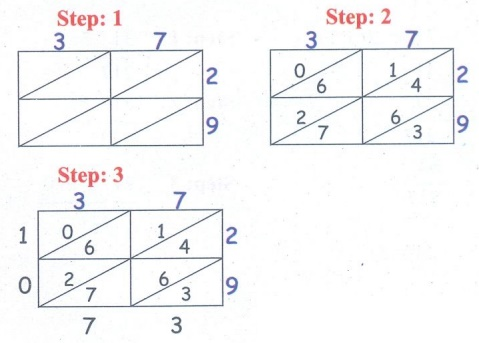Home | | Maths 4th Std | Exercise 2.1 (Multiplication by lattice algorithm)

# Exercise 2.1 (Multiplication by lattice algorithm)

Text Book Back Exercises Questions with Answers, Solution : 4th Maths : Term 2 Unit 2 : Numbers : Exercise 2.1 (Multiplication by lattice algorithm)

Exercise 2.1

1. Multiply the following numbers by using lattice method.

(i) 52 × 49

52 x 49Answer: 52 x 49 = 2548

(ii) 347 × 5Answer: 347 x 5 = 1735

(iii) 127 × 7Answer: 127 x 7 = 889

(v) 65 × 37Answer: 65 x 37 = 2405

(v) 789 × 4Answer: 789 x 4 = 3156

(vi) 37 × 29Answer: 37 x 29 = 1073

2. Multiply the following numbers by using standard method;

(i) 72 × 29Answer: 72 × 29 = 2088

(ii) 31 × 27Answer: 31 × 27 = 837

(iii) 93 × 42Answer: 93 × 42 = 3906

(iv) 124 × 6Answer: 124 × 6 = 744

(v) 206 × 8Answer: 206 × 8 = 1648

(vi) 479 × 3

479x3Answer: 479 × 3 = 1437

3. One box contains 25 apples. How many apples are there in 36 such boxes?

No.of apples in 1 box = 25

No.of apples in 36 boxes= 36 × 25Answer: There are 900 apples in 36 boxes.

i. There are 28 pages in a daily newspaper. If Ajay buys newspapers for 45 days, how many pages will it contain?

No. of pages for 1 day = 28

No. of pages for 45 days = 45 × 28Answer: It will contain 1260 pages

ii. A truck carries 125 bags of rice. If each bag has 9 kg of rice, how much kg of rice will there be in the truck.

Weight of rice in 1 bag = 9 kg

Weight of rice in 125 bags = 125 × 9Answer: There will be 1125kg of rice in the truck.

iii. If the cost of one chair is  857. What will be the cost of 6 chairs?

Cost of 1 chair =  857

Cost of 6 chairs = 857 × 6Answer: The cost of 6 chairs will be  5142.

Tags : Numbers | Term 2 Chapter 2 | 4th Maths , 4th Maths : Term 2 Unit 2 : Numbers
Study Material, Lecturing Notes, Assignment, Reference, Wiki description explanation, brief detail
4th Maths : Term 2 Unit 2 : Numbers : Exercise 2.1 (Multiplication by lattice algorithm) | Numbers | Term 2 Chapter 2 | 4th Maths